# AngleSelection prerequisites: One of the following:

Three points

Two straight objects with a common endpoint, optionally including the common endpoint

One or more angle markers

This Measure menu command measures an angle.

If you select three points, the first selected point defines the initial side of the angle, the second point defines the vertex, and the third point defines the terminal side of the angle.

Be sure to select the vertex point as the second point.

If you select two straight objects that share an endpoint, the first straight object defines the initial side and the second defines the terminal side. You can select the common vertex or not, as you prefer.

It’s often convenient to use a selection rectangle to select both straight objects and their common vertex.

If you select an angle marker, the initial and final sides are determined by the angle marker.

This table shows how the possible valuse of an angle measurement depend on the angle units chosen on the Units panel of the Preferences dialog box.

 Angle Units (set in Preferences) Selected Object(s) Example Degrees Directed Degrees Radians Three points or two straight objects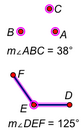0° ≤ θ ≤ 180° –180° < θ ≤ 180° –π < θ ≤ π Simple Angle Marker: A simple angle is always less than or equal to a straight angle. It represents the smallest turn at the vertex from the initial ray (or first point) to the terminal ray (or third point). Its direction can be counter-clockwise or clockwise.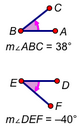0° ≤ θ ≤ 180° –180° < θ ≤ 180° –π < θ ≤ π Reflex: A reflex angle is always greater than or equal to a straight angle. It represents the greatest turn at the vertex from the initial ray (or first point) to the terminal ray (or third point). Its direction can be counter-clockwise or clockwise.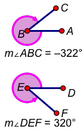180° ≤ θ ≤ 360° 180° ≤ θ ≤ 360° or –360° < θ < –180° π  ≤ θ ≤ 2π or –2π < θ < –π Counter-clockwise: A counter-clockwise angle represents the counter-clockwise turn at the vertex from the initial ray (or first point) to the terminal ray (or third point). It can be either simple or reflex.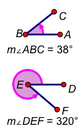0° ≤ θ < 360° 0° ≤ θ < 360° 0 ≤ θ < 2π Clockwise: A clockwise angle represents the clockwise turn at the vertex from the initial ray (or first point) to the terminal ray (or third point). It can be either simple or reflex.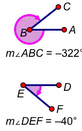0° ≤ θ < 360° –360° < θ ≤ 0° –2π < θ ≤ 0